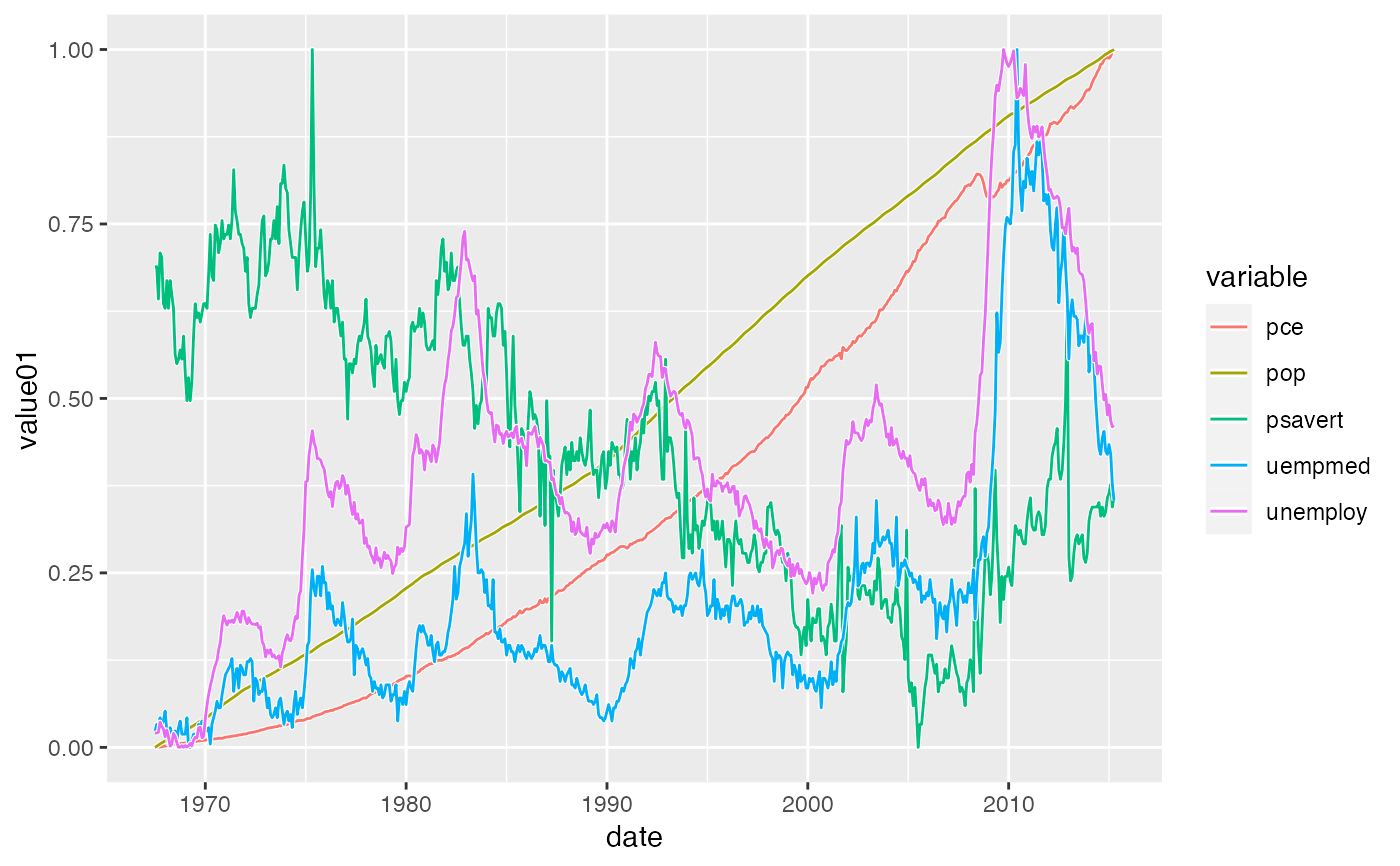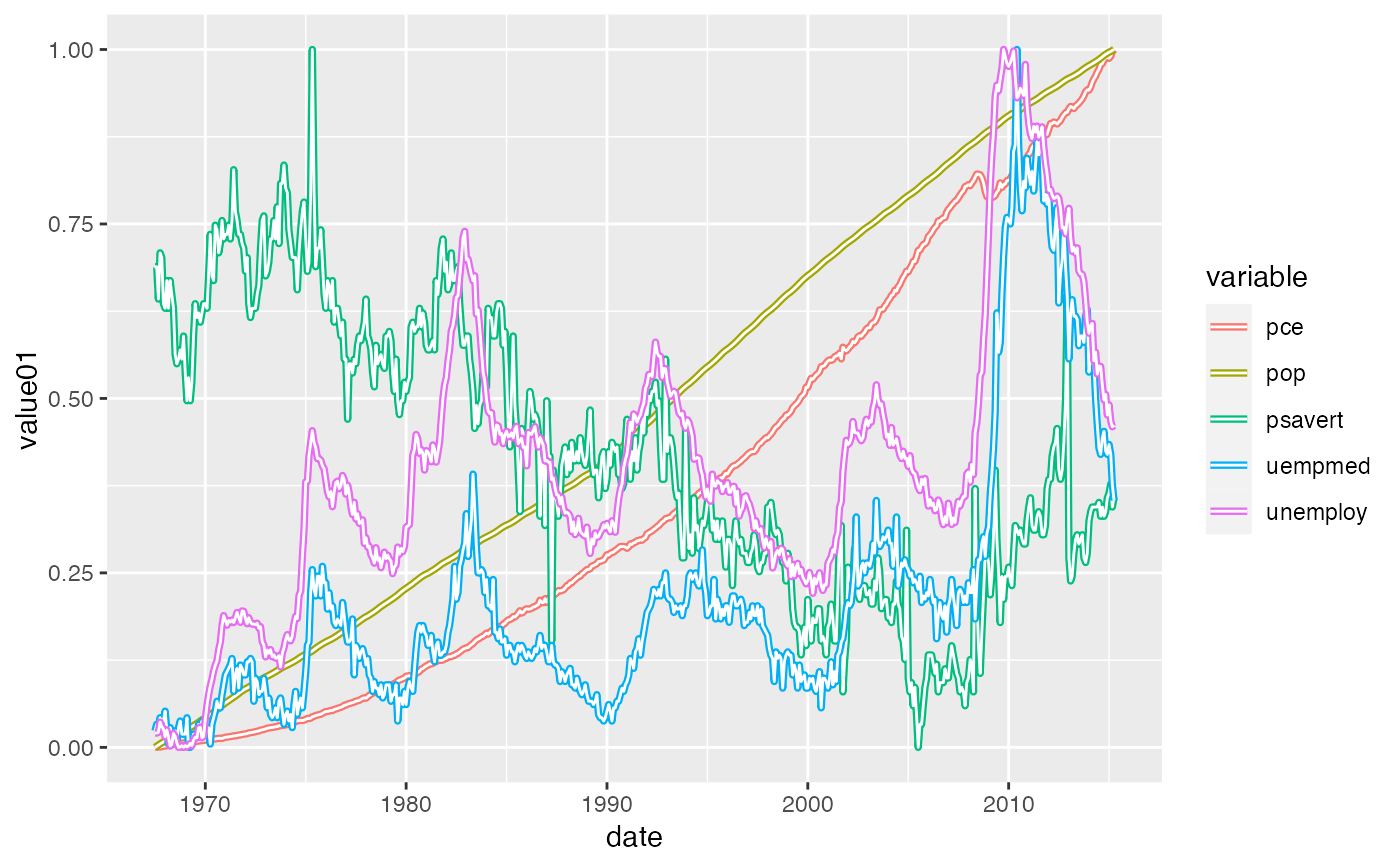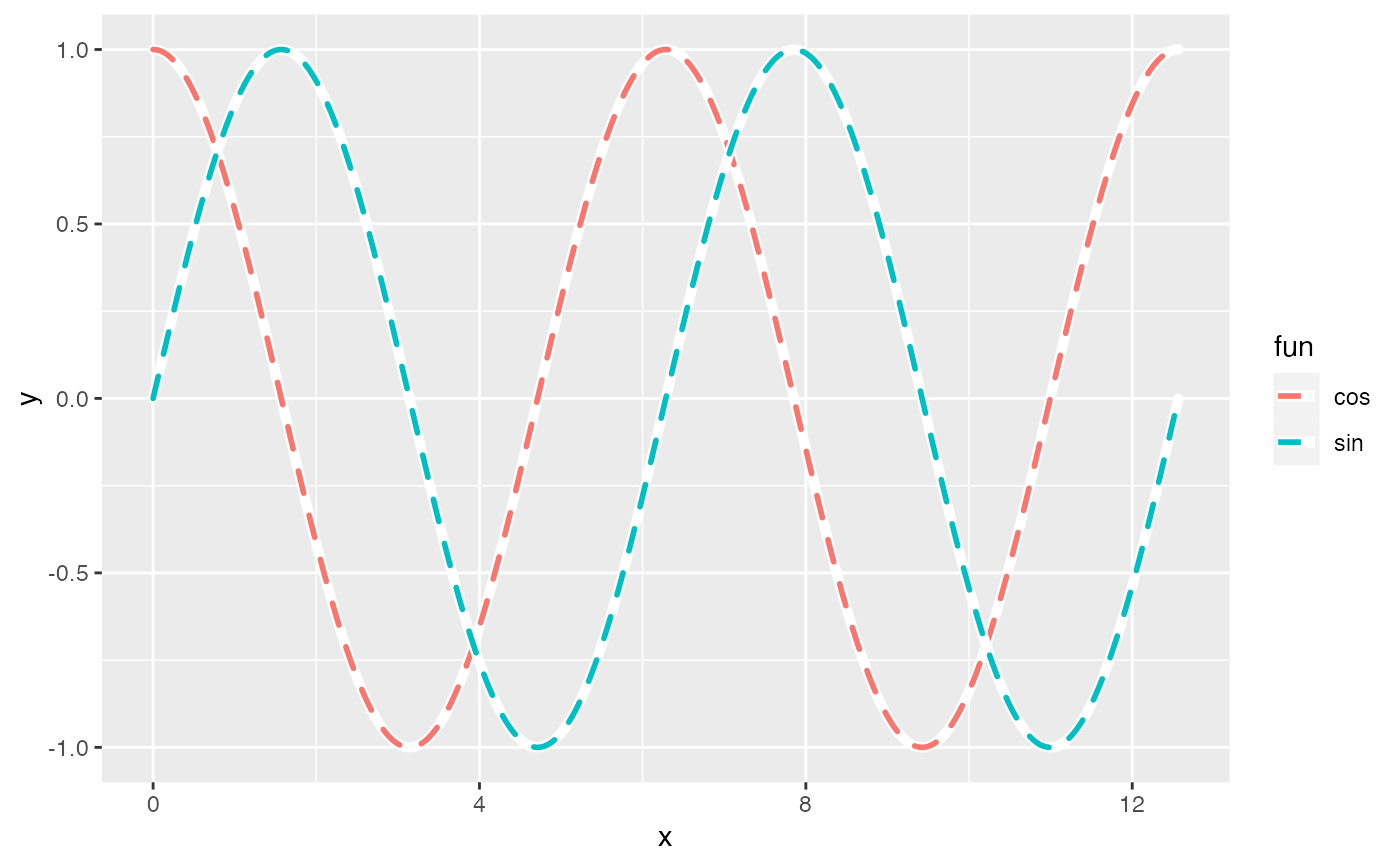This set of geoms is very similar to ggplot2::geom_path(), ggplot2::geom_line() and ggplot2::geom_step(), with the only difference being that they accept two additional aesthetics, bordercolour and bordersize. For additional documentation, please refer to the ggplot2 geoms.

geom_borderpath(
mapping = NULL,
data = NULL,
stat = "identity",
position = "identity",
...,
lineend = "butt",
linejoin = "round",
linemitre = 10,
arrow = NULL,
na.rm = FALSE,
show.legend = NA,
inherit.aes = TRUE
)

geom_borderline(
mapping = NULL,
data = NULL,
stat = "identity",
position = "identity",
...,
lineend = "butt",
linejoin = "round",
linemitre = 10,
arrow = NULL,
na.rm = FALSE,
show.legend = NA,
inherit.aes = TRUE
)

geom_borderstep(
mapping = NULL,
data = NULL,
stat = "identity",
position = "identity",
direction = "hv",
na.rm = FALSE,
show.legend = NA,
inherit.aes = TRUE,
...
)

## Arguments

mapping Set of aesthetic mappings created by aes() or aes_(). If specified and inherit.aes = TRUE (the default), it is combined with the default mapping at the top level of the plot. You must supply mapping if there is no plot mapping. The data to be displayed in this layer. There are three options: If NULL, the default, the data is inherited from the plot data as specified in the call to ggplot(). A data.frame, or other object, will override the plot data. All objects will be fortified to produce a data frame. See fortify() for which variables will be created. A function will be called with a single argument, the plot data. The return value must be a data.frame, and will be used as the layer data. A function can be created from a formula (e.g. ~ head(.x, 10)). The statistical transformation to use on the data for this layer, as a string. Position adjustment, either as a string, or the result of a call to a position adjustment function. Other arguments passed on to layer(). These are often aesthetics, used to set an aesthetic to a fixed value, like colour = "red" or size = 3. They may also be parameters to the paired geom/stat. Line end style (round, butt, square). Line join style (round, mitre, bevel). Line mitre limit (number greater than 1). Arrow specification, as created by grid::arrow(). If FALSE, the default, missing values are removed with a warning. If TRUE, missing values are silently removed. logical. Should this layer be included in the legends? NA, the default, includes if any aesthetics are mapped. FALSE never includes, and TRUE always includes. It can also be a named logical vector to finely select the aesthetics to display. If FALSE, overrides the default aesthetics, rather than combining with them. This is most useful for helper functions that define both data and aesthetics and shouldn't inherit behaviour from the default plot specification, e.g. borders(). direction of stairs: 'vh' for vertical then horizontal, 'hv' for horizontal then vertical, or 'mid' for step half-way between adjacent x-values.

## Value

A ggproto layer object

## Examples

require(ggplot2)
# geom_borderline() adds a border around lines
ggplot(economics_long, aes(date, value01, colour = variable)) +
geom_borderline()# You can control the size and colour of the border with the
# bordersize and bordercolour aesthetics:
ggplot(economics_long, aes(date, value01, bordercolour = variable)) +
geom_borderline(bordersize = .4, colour = "white")# The background 'border' part of the geom is always solid, however this
# can be used to create some nice effects:
x <- seq(0, 4 * pi, length.out = 500)
test_data <- data.frame(
x = rep(x, 2), y = c(sin(x), cos(x)),
fun = rep(c("sin", "cos"), each = 500)
)
ggplot(test_data, aes(x, y, colour = fun)) +
geom_borderline(size = 1, linetype = "dashed", lineend = "round")